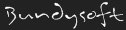L3DT documentationLarge 3D terrain generator

# Variable Data Types

Most data in Zeolite is managed by dynamically-typed variables, represented by ZVAR handles, and manipulated using the set of functions listed on the function reference page.

The tables below present the list of valid variable types (including classes) by their 'token' (defined in the `Zeolite_defines.h`), the numeric value of that token, and by the string returned by the ztype_GetName function.

## Basic types

Name string Token VarID Description
[NULL] VarID_INVALID 0 Used for invalid variable handles, or uninitialised variables.
“void” VarID_void 1 A variable with no data (but has a name).
“variant” VarID_variant 2 A variable whose value is another variable, the type of which is free to change. This data type is and should only be used for variable input extension functions.
“bool” VarID_bool 3 Boolean value. Data is a C/C++ 'bool' type.
“char” VarID_char 4 8-bit signed integer and/or ASCII character.
“byte” VarID_byte 5 8-bit unsigned integer.
“ubyte” VarID_ubyte 5 Same as 'byte'.
“sbyte” VarID_sbyte 104 8-bit signed integer.
“short” VarID_short 6 16-bit signed integer.
“ushort” VarID_ushort 105 16-bit unsigned integer.
“int” VarID_int 7 32-bit signed integer.
“uint” VarID_uint 106 32-bit unsigned integer.
“int64” VarID_int64 8 64-bit integer.
“float” VarID_float 9 32-bit floating-point variable.
“double” VarID_double 10 64-bit floating-point variable.
“colour” VarID_colour 13 24-bit colour variable. Data is a ColourPixel struct.
“vector3c” VarID_vector3c 101 A three-component 8-bit signed integer vector. Data is a vector3c struct.
“vector3i” VarID_vector3i 102 A three-component 32-bit signed integer vector. Data is a vector3i struct.
“vector3f” VarID_vector3f 14 A three-component floating-point vector. Data is a vector3f struct.
“vector3d” VarID_vector3d 103 A three-component double-precision floating-point vector. Data is a vector3d struct.
“vector4c” VarID_vector4c 107 A four-component 8-bit signed integer vector. Data is a vector4c struct.
“vector4i” VarID_vector4i 108 A four-component 32-bit signed integer vector. Data is a vector4i struct.
“vector4f” VarID_vector4f 109 A four-component floating-point vector. Data is a vector4f struct.
“vector4d” VarID_vector4d 110 A four-component double-precision floating-point vector. Data is a vector4d struct.
“matrix3f” VarID_matrix3f 111 A 3×3-component single precision floating point matrix. Data is a matrix3f struct.
“voidptr” VarID_voidptr 20 Memory address (32 bit). Data is a C/C++ 'void*' type.
“varid” VarID_varid 23 A 32-bit integer, containing one of the VarID values enumerated in this table.
“hvar” VarID_hvar 25 A variable handle. Data is a ZVAR handle to another variable.

## Classes

Name string Token VarID Wrapper class Description
“map” VarID_map 60 CzMap Map variable.
“varlist” VarID_varlist 31 CzList List variable.
“string” VarID_string 11 CzStr String variable.
“format” VarID_format 100 CzFormat File format variable.
“buffer” VarID_buffer 15 CzBuffer Buffer variable.
“filesel” VarID_filesel 300 CzFileSel A file open/save dialog box with filter options, default directories, etc.
“dirsel” VarID_dirsel 301 CzDirSel Dialog box by which users can browse for a directory of the file system.
“combosel” VarID_combosel 302 CzComboSel Dialog box by which users can select from a list of options.
“climate” VarID_climate 340 CzClimate Climate variable.
“landtype” VarID_landtype 341 CzLandType LandType variable.
“progbox” VarID_progbox 600 CzProgBox Dialog box for displaying progress.
“zeofunc” VarID_zeofunc 510 CzFunc Wrapper for a Zeolite extension function.